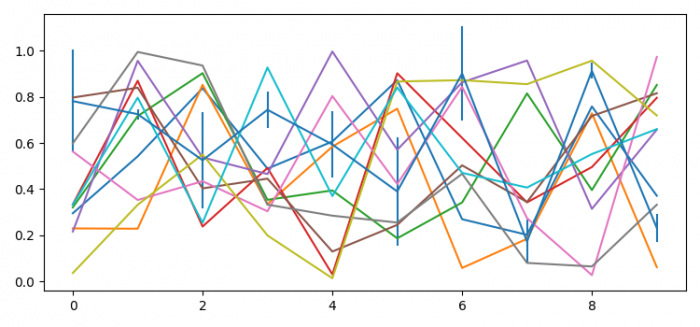# How to force errorbars to render last with Matplotlib?

To force errorbars to render last with matplotlib, we can take the following steps −

• Set the figure size and adjust the padding between and around the subplots.
• Create a new figure or activate an existing figure using figure() method.
• Get the current axis using gca() method.
• Plot the list of lines
• Plot y versus x as lines and/or markers with attached errorbars.
• To display the figure, use show() method.

## Example

import matplotlib.pyplot as plt
import numpy as np

plt.rcParams["figure.figsize"] = [7.50, 3.50]
plt.rcParams["figure.autolayout"] = True

fig = plt.figure()
ax = plt.gca()

[ax.plot(np.random.rand(10)) for j in range(10)]
ax.errorbar(range(10), np.random.rand(10), yerr=.3 * np.random.rand(10))

plt.show()

## Output